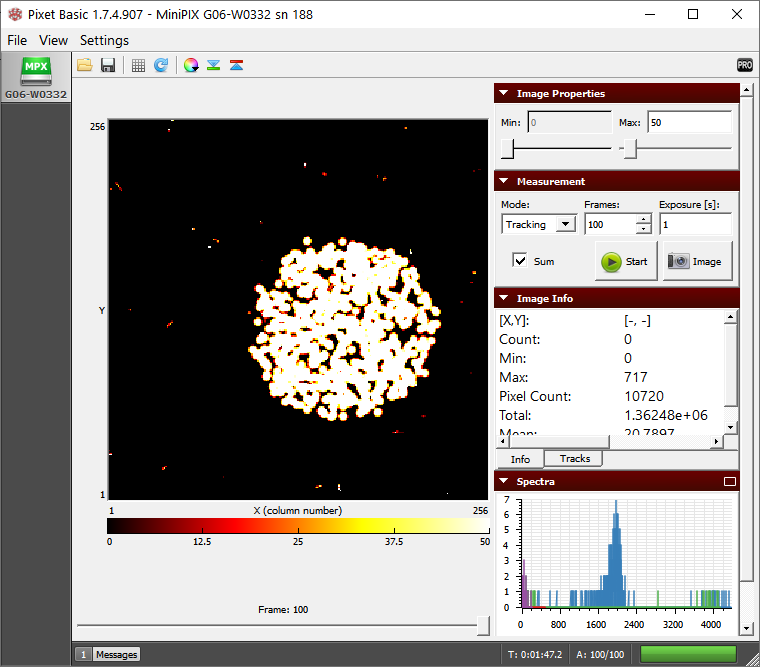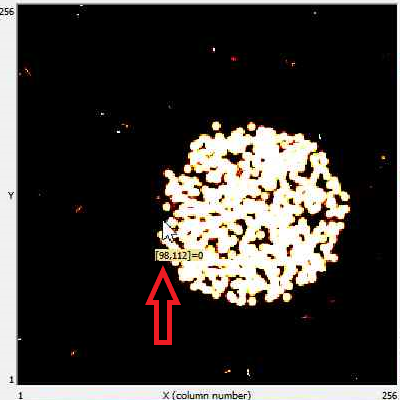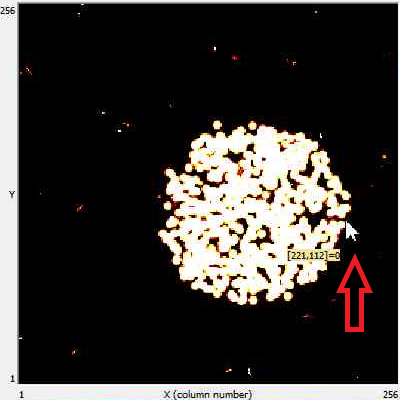# Particle Beam Collimation of Alpha Particles

## Equipment

Stage, camera holder, source holder, collimator, polonium-210, Minipix-EDU

## Procedure

1. Launch the Pixet Basic software and modify settings to the following:
1. Min Level: 0
2. Max Level: 50
3. Measurement Mode: Tracking
4. Frames: 100
5. Exposure: 1 s
6. Sum: check
7. Color Map: Hot
2. Mount the MiniPix EDU camera and polonium on the Stage. Make sure to put polonium in the rear slot and then insert the collimator.
3. Keep the camera and the source at a distance of 2.5 cm and click on the play button.

## Results

1. We observe a circular image of concentrated alpha particles (Fig. 1).Figure 1. The collimated beam of alpha particles from polonium-210

2. We can determine the diameter of the circle hovering the cursor to the two extreme opposite ends of the circle. Making sure the y-coordinate for the two points are the same, subtract the x- coordinate of one point from another as shown in figure 2(a) and 2(b).

221- 98 = 123 pixelsa) x-coordinate of the left corner b) x-coordinate of the right corner
Figure 2. Hovering the cursor to measure the diameter

3. The difference gives us the number of pixels and since each pixel is 55 µm x 55 µm, the diameter will be

123 x 55 = 6765 µm = 6.76 mm

4. The diameter of the collimator is 6 mm and that of the observed circle of alpha particles is 6.76 mm. The error is ~12.7% which is quite low and it can be reduced if we further decrease the distance between the collimator and camera.
5. The data collected with the minimum distance resulted in only 1.7% of error.
Caution: It is not advisable to perform the scan at less than 2.5 cm distance because there is a high risk of hitting the collimator into the camera sensor.

## Conclusion

1. The observed circle of alpha particles is proof of the linear propagation of particles.
2. Also, the increase in diameter is proof of the linear divergent beam of alpha particles.